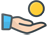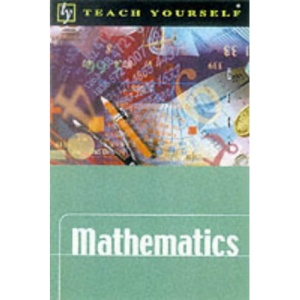30 Day Refund Guarantee# Mathematics (Teach Yourself)

by TrevorJohnson (Author), HughNeill (Author)

##### Synopsis

This step-by-step introduction offers clear explanations and worked examples that should guide the reader to an understanding of essential mathematical concepts and techniques. Throughout the book exercises (with answers) are provided to test and reinforce the reader's understanding of the topic. The book covers: the basic arithmetical processes, algebra and geometry; problems involving percentages, profit and loss and interest calculations; expressing numbers as the products of prime factors; Pythagoras' theorem and trigonometry; fractions and decimals; organizing and illustrating data; and using ratio and proportion.

Save:\$7.92 (71%)

Quantity

2 in stock